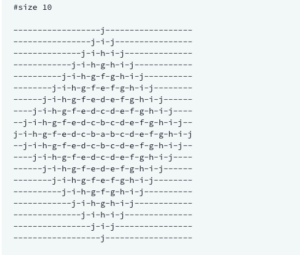You are given an integer, N . Your task is to print an alphabet rangoli of size N. (Rangoli is a form of Indian folk art based on creation of patterns.)
Different sizes of alphabet rangoli are shown below:Go to problem statement.
Explanation Video:

Mentor: Satyendra Jaiswal
Langauge: Python

## Source Code: print_rangoli solution

``````
def print_rangoli(size):
n=size
l1=list(map(chr,range(97,123)))
x=l1[n-1::-1]+l1[1:n]
m=len('-'.join(x))
for i in range(1,n):
print('-'.join(l1[n-1:n-i:-1]+l1[n-i:n]).center(m,'-'))
for i in range(n,0,-1):
print('-'.join(l1[n-1:n-i:-1]+l1[n-i:n]).center(m,'-'))

if __name__ == '__main__':
n = int(input())
print_rangoli(n)
``````